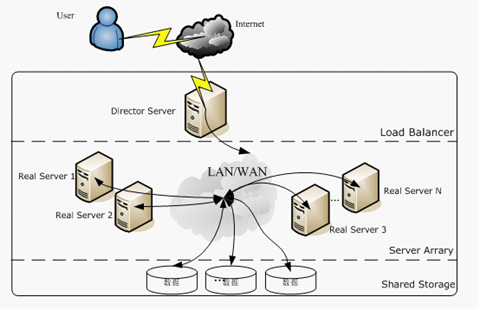分类算法评估指标

Confusion Matrix 混淆矩阵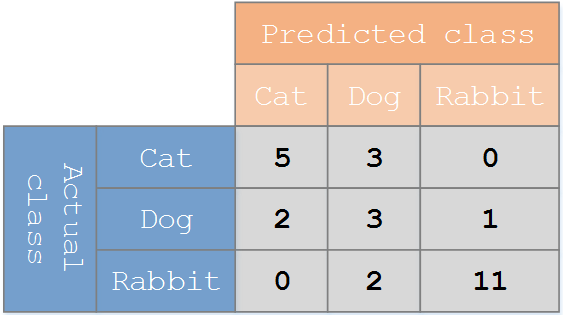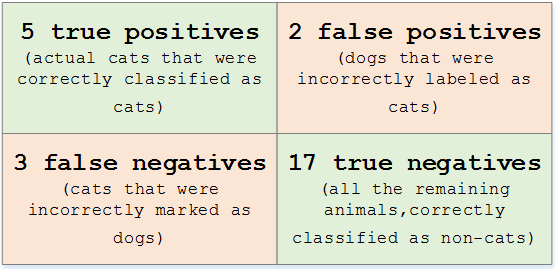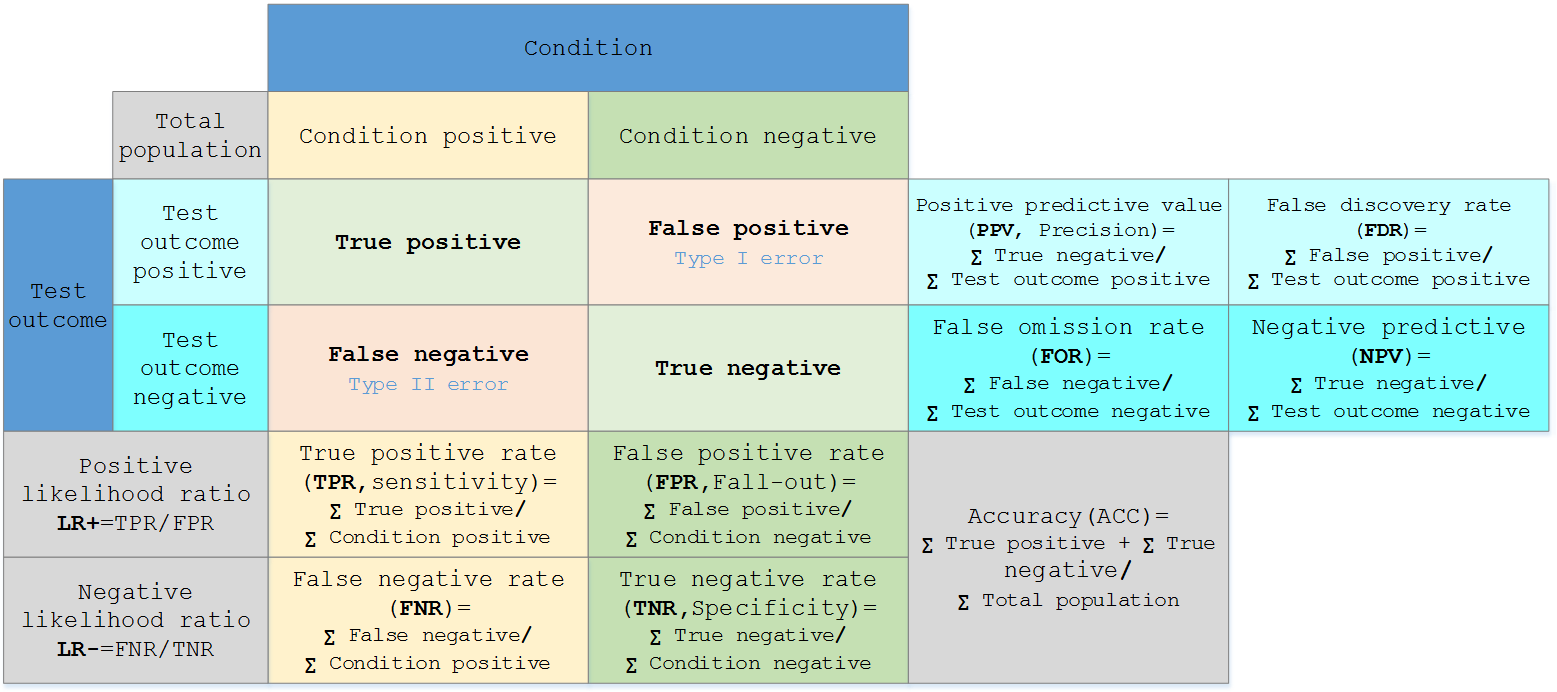knn = KNeighborsClassifier(n_neighbors=7)
knn.fit(X_train,y_train)

from sklearn.metrics import confusion_matrix
y_pred = knn.predict(X_test)
confusion_matrix(y_test,y_pred)
pd.crosstab(y_test, y_pred, rownames=['True'], colnames=['Predicted'], margins=True)
Predicted    0   1  All
True
0          165  36  201
1           47  60  107
All        212  96  308

Classification Report

sklearn中的classification_report函数用于显示主要分类指标的文本报告。在报告中显示每个类的精确度，召回率，F1值等信息。

• y_true：1维数组，或标签指示器数组/稀疏矩阵，目标值。
• y_pred：1维数组，或标签指示器数组/稀疏矩阵，分类器返回的估计值。
• labels：array，shape = [n_labels]，报表中包含的标签索引的可选列表。
• target_names：字符串列表，与标签匹配的可选显示名称（相同顺序）。
• sample_weight：类似于shape = [n_samples]的数组，可选项，样本权重。
• digits：int，输出浮点值的位数。
from sklearn.metrics import classification_report
print(classification_report(y_test,y_pred))
precision    recall  f1-score   support
0       0.78      0.82      0.80       201
1       0.62      0.56      0.59       107
avg / total       0.73      0.73      0.73       308

精确度＆召回率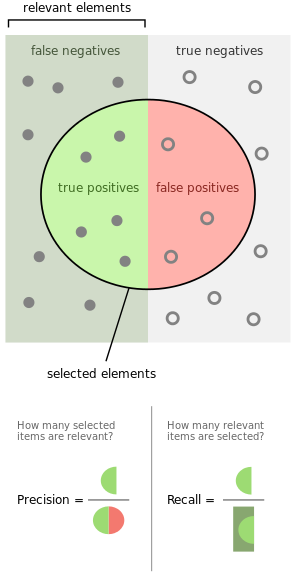from sklearn.metrics import precision_score
from sklearn.metrics import recall_score

print(precision_score(y_test, y_pred))
print(recall_score(y_test, y_pred))

F1值

$$F = \frac{(a^2+1)P\times R}{a^2(P+R)}$$

$$F = \frac{2PR}{P+R}$$

ROC曲线和AUC值

ROC

• TP：正确肯定的数目
• FN：漏报，没有正确找到的匹配的数目
• FP：误报，给出的匹配是不正确的
• TN：正确拒绝的非匹配对数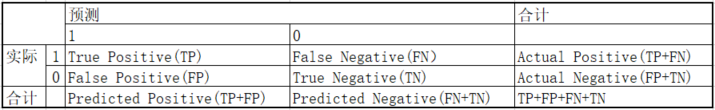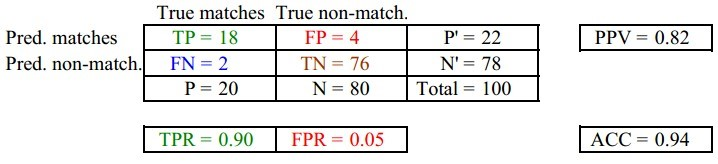• FPR = FP/(FP + TN) 负样本中的错判率（假警报率）
• TPR = TP/(TP + FN) 判对样本中的正样本率（命中率）
• ACC = (TP + TN) / (P+N) 判对准确率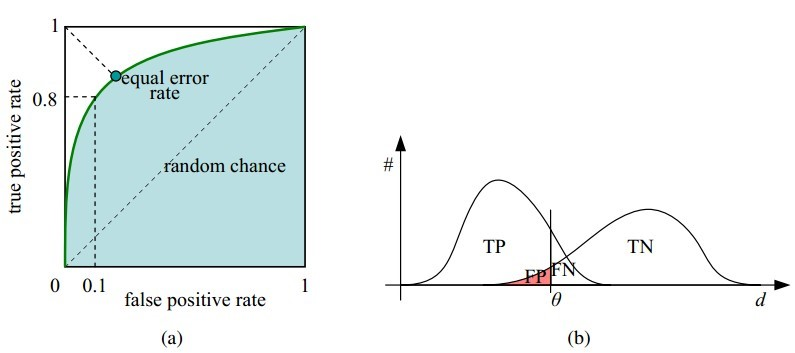ROC曲线和它相关的比率

• 理想情况下，TPR应该接近1，FPR应该接近0。ROC曲线上的每一个点对应于一个threshold，对于一个分类器，每个threshold下会有一个TPR和FPR。比如Threshold最大时，TP=FP=0，对应于原点；Threshold最小时，TN=FN=0，对应于右上角的点(1,1)。
• P和N得分不作为特征间距离d的一个函数，随着阈值theta增加，TP和FP都增加。
• 横轴FPR：1-TNR，1-Specificity，FPR越大，预测正类中实际负类越多。
• 纵轴TPR：Sensitivity(正类覆盖率)，TPR越大，预测正类中实际正类越多。
• 理想目标：TPR=1，FPR=0，即图中(0,1)点，故ROC曲线越靠拢(0,1)点，越偏离45度对角线越好，Sensitivity、Specificity越大效果越好。
• 随着阈值threshold调整，ROC坐标系里的点如何移动可以参考：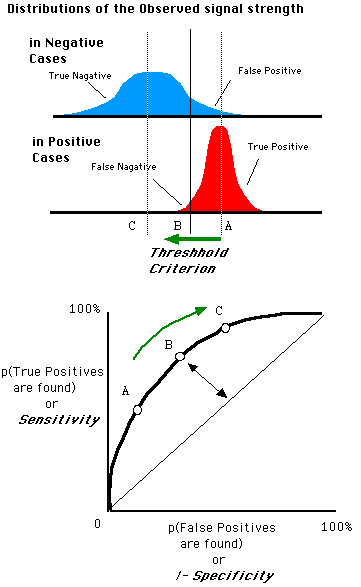In signal detection theory, a receiver operating characteristic (ROC), or simply ROC curve, is a graphical plot which illustrates the performance of a binary classifier system as its discrimination threshold is varied.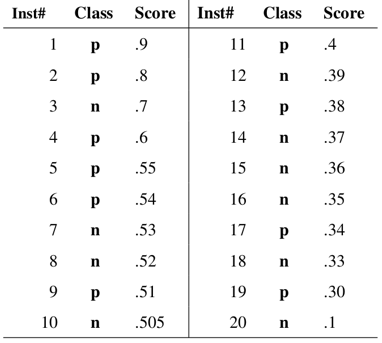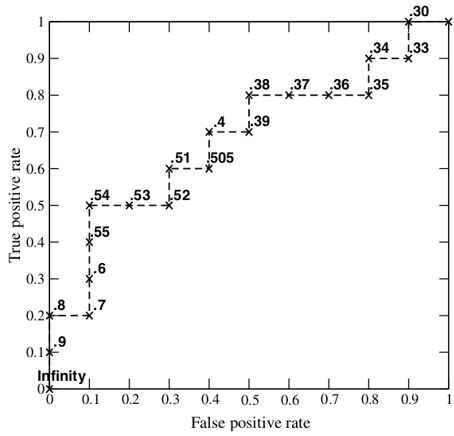from sklearn.metrics import roc_curve

y_pred_proba = knn.predict_proba(X_test)[:,1]
fpr, tpr, thresholds = roc_curve(y_test, y_pred_proba)
plt.plot([0,1],[0,1],'k--')
plt.plot(fpr,tpr, label='Knn')
plt.xlabel('fpr')
plt.ylabel('tpr')
plt.title('Knn(n_neighbors=7) ROC curve')
plt.show()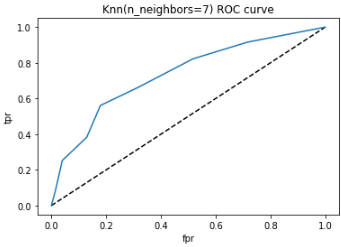AUC

ROC曲线是根据与那条参照线进行比较来判断模型的好坏，但这只是一种直觉上的定性分析，如果我们需要精确一些，就要用到AUC，也就是ROC曲线下面积。其判定方法是AUC应该大于0.5。如图所示：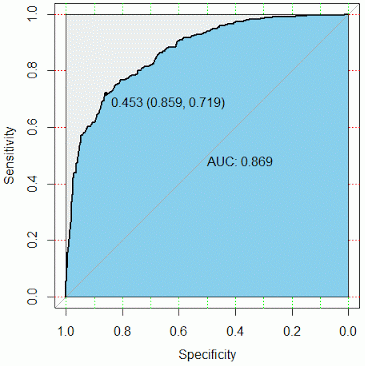from sklearn.metrics import roc_auc_score
roc_auc_score(y_test,y_pred_proba)
0.7345050448691124

对数损失（LogLoss）

$$LogLoss = – \frac{1}{n}\sum_{i}[y_i\log(y_i^{‘})+(1-y_i)\log(1-y_i^{‘})]$$

LogLoss衡量的是预测概率分布和真实概率分布的差异性，取值越小越好。

logloss和auc的区别：

• logloss主要是评估是否准确的，auc是用来评估是把正样本排到前面的能力，评估的方面不一样。
• logloss主要评估整体准确性，多用于数据平衡的情况。auc用来评估数据不平衡情况的，模型准确度。如果是平衡的分类问题，那么AUC和LogLoss都可以。

GridSearchCV 网格搜索

GridSearchCV，它存在的意义就是自动调参，只要把参数输进去，就能给出最优化的结果和参数。但是这个方法适合于小数据集，一旦数据的量级上去了，很难得出结果。这个时候就是需要动脑筋了。数据量比较大的时候可以使用一个快速调优的方法——坐标下降。它其实是一种贪心算法：拿当前对模型影响最大的参数调优，直到最优化；再拿下一个影响最大的参数调优，如此下去，直到所有的参数调整完毕。这个方法的缺点就是可能会调到局部最优而不是全局最优，但是省时间省力，巨大的优势面前，还是试一试吧，后续可以再拿bagging再优化。回到sklearn里面的GridSearchCV，GridSearchCV用于系统地遍历多种参数组合，通过交叉验证确定最佳效果参数。

from sklearn.model_selection import GridSearchCV

param_grid = {'n_neighbors':np.arange(1,50)}
knn = KNeighborsClassifier()
knn_cv= GridSearchCV(knn,param_grid,cv=5)
knn_cv.fit(X,y)
print(knn_cv.best_score_)
print(knn_cv.best_params_)
0.7578125
{'n_neighbors': 14}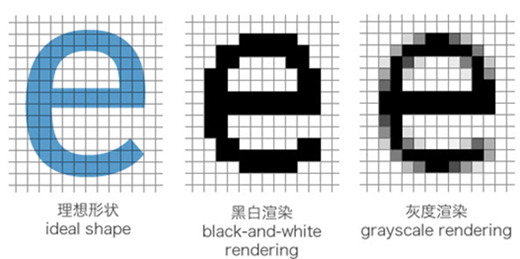网站与APP开发中的字体设置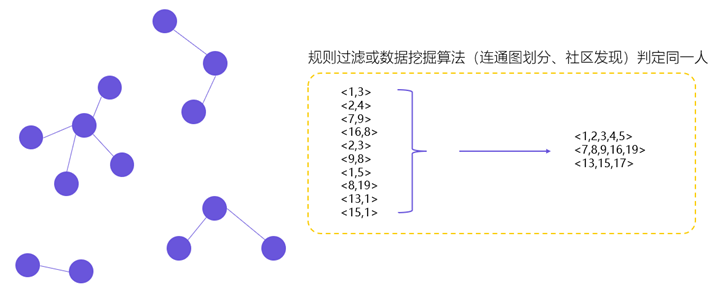用户体系搭建之ID-Mapping